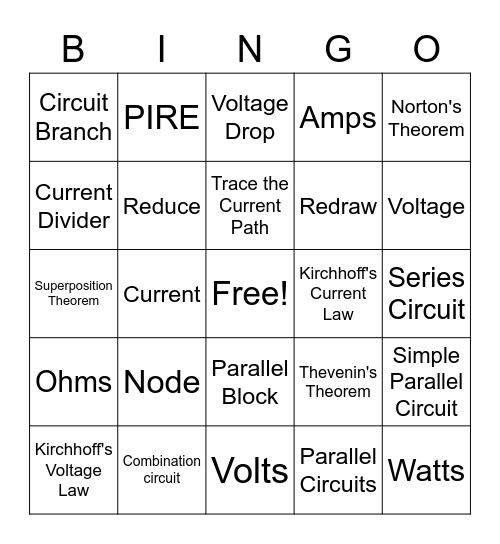# Electricity BingoThis bingo card has a free space and 24 words: Node, Combination circuit, Trace the Current Path, Kirchhoff's Voltage Law, Norton's Theorem, Circuit Branch, Current Divider, PIRE, Kirchhoff's Current Law, Parallel Block, Redraw, Superposition Theorem, Thevenin's Theorem, Simple Parallel Circuit, Reduce, Parallel Circuits, Series Circuit, Voltage Drop, Voltage, Current, Watts, Ohms, Amps and Volts.

## Play Online